If you're running AdBlock, please consider whitelisting this site if you'd like to support LearnOpenGL; and no worries, I won't be mad if you don't :)

# Theory

PBR, or more commonly known as physically based rendering, is a collection of render techniques that are more or less based on the same underlying theory that more closely matches that of the physical world. As physically based rendering aims to mimic light in a physically plausible way, it generally looks more realistic compared to our original lighting algorithms like Phong and Blinn-Phong. Not only does it look better, as it closely approximates actual physics, we (and especially the artists) can author surface materials based on physical parameters without having to resort to cheap hacks and tweaks to make the lighting look right. One of the bigger advantages of authoring materials based on physical parameters is that these materials will look correct regardless of lighting conditions; something that is not true in non-PBR pipelines.

Physically based rendering is still nonetheless an approximation of reality (based on the principles of physics) which is why it's not called physical shading, but physically based shading. For a PBR lighting model to be considered physically based, it has to satisfy the following 3 conditions (don't worry, we'll get to them soon enough):

1. Be based on the microfacet surface model.
2. Be energy conserving.
3. Use a physically based BRDF.

In the next PBR chapters we'll be focusing on the PBR approach as originally explored by Disney and adopted for real-time display by Epic Games. Their approach, based on the metallic workflow, is decently documented, widely adopted on most popular engines, and looks visually amazing. By the end of these chapters we'll have something that looks like this: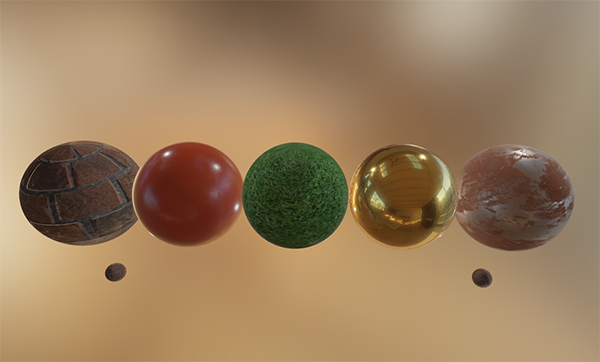Keep in mind, the topics in these chapters are rather advanced so it is advised to have a good understanding of OpenGL and shader lighting. Some of the more advanced knowledge you'll need for this series are: framebuffers, cubemaps, gamma correction, HDR, and normal mapping. We'll also delve into some advanced mathematics, but I'll do my best to explain the concepts as clear as possible.

## The microfacet model

All the PBR techniques are based on the theory of microfacets. The theory describes that any surface at a microscopic scale can be described by tiny little perfectly reflective mirrors called microfacets. Depending on the roughness of a surface, the alignment of these tiny little mirrors can differ quite a lot:The rougher a surface is, the more chaotically aligned each microfacet will be along the surface. The effect of these tiny-like mirror alignments is, that when specifically talking about specular lighting/reflection, the incoming light rays are more likely to scatter along completely different directions on rougher surfaces, resulting in a more widespread specular reflection. In contrast, on a smooth surface the light rays are more likely to reflect in roughly the same direction, giving us smaller and sharper reflections:No surface is completely smooth on a microscopic level, but seeing as these microfacets are small enough that we can't make a distinction between them on a per-pixel basis, we statistically approximate the surface's microfacet roughness given a roughness parameter. Based on the roughness of a surface, we can calculate the ratio of microfacets roughly aligned to some vector $$h$$. This vector $$h$$ is the halfway vector that sits halfway between the light $$l$$ and view $$v$$ vector. We've discussed the halfway vector before in the advanced lighting chapter which is calculated as the sum of $$l$$ and $$v$$ divided by its length:

$h = \frac{l + v}{\|l + v\|}$

The more the microfacets are aligned to the halfway vector, the sharper and stronger the specular reflection. Together with a roughness parameter that varies between 0 and 1, we can statistically approximate the alignment of the microfacets: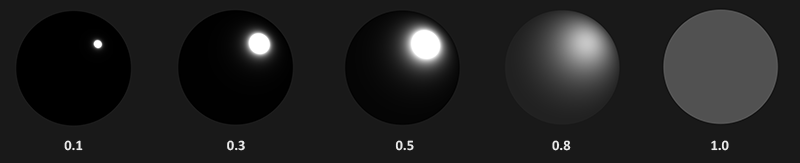We can see that higher roughness values display a much larger specular reflection shape, in contrast with the smaller and sharper specular reflection shape of smooth surfaces.

## Energy conservation

The microfacet approximation employs a form of energy conservation: outgoing light energy should never exceed the incoming light energy (excluding emissive surfaces). Looking at the above image we see the specular reflection area increase, but also its brightness decrease at increasing roughness levels. If the specular intensity were to be the same at each pixel (regardless of the size of the specular shape) the rougher surfaces would emit much more energy, violating the energy conservation principle. This is why we see specular reflections more intensely on smooth surfaces and more dimly on rough surfaces.

For energy conservation to hold, we need to make a clear distinction between diffuse and specular light. The moment a light ray hits a surface, it gets split in both a refraction part and a reflection part. The reflection part is light that directly gets reflected and doesn't enter the surface; this is what we know as specular lighting. The refraction part is the remaining light that enters the surface and gets absorbed; this is what we know as diffuse lighting.

There are some nuances here as refracted light doesn't immediately get absorbed by touching the surface. From physics, we know that light can be modeled as a beam of energy that keeps moving forward until it loses all of its energy; the way a light beam loses energy is by collision. Each material consists of tiny little particles that can collide with the light ray as illustrated in the following image. The particles absorb some, or all, of the light's energy at each collision which is converted into heat.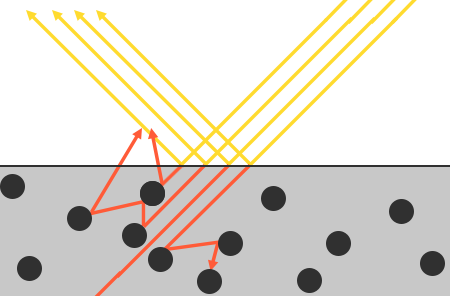Generally, not all energy is absorbed and the light will continue to scatter in a (mostly) random direction at which point it collides with other particles until its energy is depleted or it leaves the surface again. Light rays re-emerging out of the surface contribute to the surface's observed (diffuse) color. In physically based rendering however, we make the simplifying assumption that all refracted light gets absorbed and scattered at a very small area of impact, ignoring the effect of scattered light rays that would've exited the surface at a distance. Specific shader techniques that do take this into account are known as subsurface scattering techniques that significantly improve the visual quality on materials like skin, marble, or wax, but come at the price of performance.

An additional subtlety when it comes to reflection and refraction are surfaces that are metallic. Metallic surfaces react different to light compared to non-metallic surfaces (also known as dielectrics). Metallic surfaces follow the same principles of reflection and refraction, but all refracted light gets directly absorbed without scattering. This means metallic surfaces only leave reflected or specular light; metallic surfaces show no diffuse colors. Because of this apparent distinction between metals and dielectrics, they're both treated differently in the PBR pipeline which we'll delve into further down the chapter.

This distinction between reflected and refracted light brings us to another observation regarding energy preservation: they're mutually exclusive. Whatever light energy gets reflected will no longer be absorbed by the material itself. Thus, the energy left to enter the surface as refracted light is directly the resulting energy after we've taken reflection into account.

We preserve this energy conserving relation by first calculating the specular fraction that amounts the percentage the incoming light's energy is reflected. The fraction of refracted light is then directly calculated from the specular fraction as:


float kS = calculateSpecularComponent(...); // reflection/specular fraction
float kD = 1.0 - kS;                        // refraction/diffuse  fraction


This way we know both the amount the incoming light reflects and the amount the incoming light refracts, while adhering to the energy conservation principle. Given this approach, it is impossible for both the refracted/diffuse and reflected/specular contribution to exceed 1.0, thus ensuring the sum of their energy never exceeds the incoming light energy. Something we did not take into account in the previous lighting chapters.

## The reflectance equation

This brings us to something called the render equation, an elaborate equation some very smart folks out there came up with that is currently the best model we have for simulating the visuals of light. Physically based rendering strongly follows a more specialized version of the render equation known as the reflectance equation. To properly understand PBR, it's important to first build a solid understanding of the reflectance equation:

$L_o(p,\omega_o) = \int\limits_{\Omega} f_r(p,\omega_i,\omega_o) L_i(p,\omega_i) n \cdot \omega_i d\omega_i$

The reflectance equation appears daunting at first, but as we'll dissect it you'll see it slowly starts to makes sense. To understand the equation, we have to delve into a bit of radiometry. Radiometry is the measurement of electromagnetic radiation, including visible light. There are several radiometric quantities we can use to measure light over surfaces and directions, but we will only discuss a single one that's relevant to the reflectance equation known as radiance, denoted here as $$L$$. Radiance is used to quantify the magnitude or strength of light coming from a single direction. It's a bit tricky to understand at first as radiance is a combination of multiple physical quantities so we'll focus on those first:

Radiant flux: radiant flux $$\Phi$$ is the transmitted energy of a light source measured in Watts. Light is a collective sum of energy over multiple different wavelengths, each wavelength associated with a particular (visible) color. The emitted energy of a light source can therefore be thought of as a function of all its different wavelengths. Wavelengths between 390nm to 700nm (nanometers) are considered part of the visible light spectrum i.e. wavelengths the human eye is able to perceive. Below you'll find an image of the different energies per wavelength of daylight: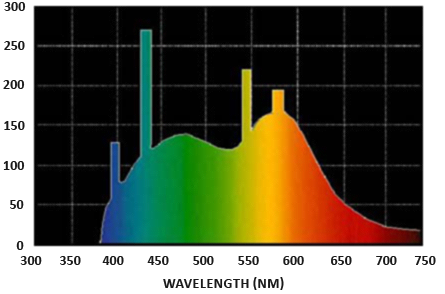The radiant flux measures the total area of this function of different wavelengths. Directly taking this measure of wavelengths as input is slightly impractical so we often make the simplification of representing radiant flux, not as a function of varying wavelength strengths, but as a light color triplet encoded as RGB (or as we'd commonly call it: light color). This encoding does come at quite a loss of information, but this is generally negligible for visual aspects.

Solid angle: the solid angle, denoted as $$\omega$$, tells us the size or area of a shape projected onto a unit sphere. The area of the projected shape onto this unit sphere is known as the solid angle; you can visualize the solid angle as a direction with volume: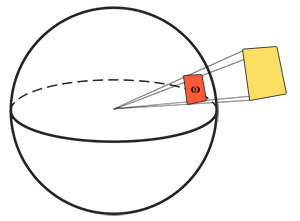Think of being an observer at the center of this unit sphere and looking in the direction of the shape; the size of the silhouette you make out of it is the solid angle.

Radiant intensity: radiant intensity measures the amount of radiant flux per solid angle, or the strength of a light source over a projected area onto the unit sphere. For instance, given an omnidirectional light that radiates equally in all directions, the radiant intensity can give us its energy over a specific area (solid angle):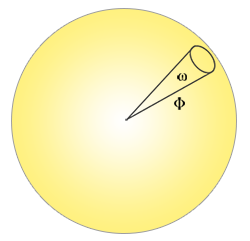The equation to describe the radiant intensity is defined as follows:

$I = \frac{d\Phi}{d\omega}$

Where $$I$$ is the radiant flux $$\Phi$$ over the solid angle $$\omega$$.

With knowledge of radiant flux, radiant intensity, and the solid angle, we can finally describe the equation for radiance. Radiance is described as the total observed energy in an area $$A$$ over the solid angle $$\omega$$ of a light of radiant intensity $$\Phi$$:

$L=\frac{d^2\Phi}{ dA d\omega \cos\theta}$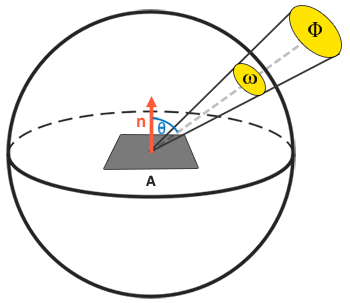Radiance is a radiometric measure of the amount of light in an area, scaled by the incident (or incoming) angle $$\theta$$ of the light to the surface's normal as $$\cos \theta$$: light is weaker the less it directly radiates onto the surface, and strongest when it is directly perpendicular to the surface. This is similar to our perception of diffuse lighting from the basic lighting chapter as $$\cos\theta$$ directly corresponds to the dot product between the light's direction vector and the surface normal:


float cosTheta = dot(lightDir, N);


The radiance equation is quite useful as it contains most physical quantities we're interested in. If we consider the solid angle $$\omega$$ and the area $$A$$ to be infinitely small, we can use radiance to measure the flux of a single ray of light hitting a single point in space. This relation allows us to calculate the radiance of a single light ray influencing a single (fragment) point; we effectively translate the solid angle $$\omega$$ into a direction vector $$\omega$$, and $$A$$ into a point $$p$$. This way, we can directly use radiance in our shaders to calculate a single light ray's per-fragment contribution.

In fact, when it comes to radiance we generally care about all incoming light onto a point $$p$$, which is the sum of all radiance known as irradiance. With knowledge of both radiance and irradiance we can get back to the reflectance equation:

$L_o(p,\omega_o) = \int\limits_{\Omega} f_r(p,\omega_i,\omega_o) L_i(p,\omega_i) n \cdot \omega_i d\omega_i$

We now know that $$L$$ in the render equation represents the radiance of some point $$p$$ and some incoming infinitely small solid angle $$\omega_i$$ which can be thought of as an incoming direction vector $$\omega_i$$. Remember that $$\cos \theta$$ scales the energy based on the light's incident angle to the surface, which we find in the reflectance equation as $$n \cdot \omega_i$$. The reflectance equation calculates the sum of reflected radiance $$L_o(p, \omega_o)$$ of a point $$p$$ in direction $$\omega_o$$ which is the outgoing direction to the viewer. Or to put it differently: $$L_o$$ measures the reflected sum of the lights' irradiance onto point $$p$$ as viewed from $$\omega_o$$.

The reflectance equation is based around irradiance, which is the sum of all incoming radiance we measure light of. Not just of a single incoming light direction, but of all incoming light directions within a hemisphere $$\Omega$$ centered around point $$p$$. A hemisphere can be described as half a sphere aligned around a surface's normal $$n$$: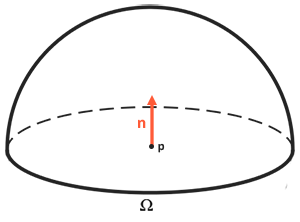To calculate the total of values inside an area or (in the case of a hemisphere) a volume, we use a mathematical construct called an integral denoted in the reflectance equation as $$\int$$ over all incoming directions $$d\omega_i$$ within the hemisphere $$\Omega$$ . An integral measures the area of a function, which can either be calculated analytically or numerically. As there is no analytical solution to both the render and reflectance equation, we'll want to numerically solve the integral discretely. This translates to taking the result of small discrete steps of the reflectance equation over the hemisphere $$\Omega$$ and averaging their results over the step size. This is known as the Riemann sum that we can roughly visualize in code as follows:


int steps = 100;
float sum = 0.0f;
vec3 P    = ...;
vec3 Wo   = ...;
vec3 N    = ...;
float dW  = 1.0f / steps;
for(int i = 0; i < steps; ++i)
{
vec3 Wi = getNextIncomingLightDir(i);
sum += Fr(P, Wi, Wo) * L(P, Wi) * dot(N, Wi) * dW;
}


By scaling the steps by dW, the sum will equal the total area or volume of the integral function. The dW to scale each discrete step can be thought of as $$d\omega_i$$ in the reflectance equation. Mathematically $$d\omega_i$$ is the continuous symbol over which we calculate the integral, and while it does not directly relate to dW in code (as this is a discrete step of the Riemann sum), it helps to think of it this way. Keep in mind that taking discrete steps will always give us an approximation of the total area of the function. A careful reader will notice we can increase the accuracy of the Riemann Sum by increasing the number of steps.

The reflectance equation sums up the radiance of all incoming light directions $$\omega_i$$ over the hemisphere $$\Omega$$ scaled by $$f_r$$ that hit point $$p$$ and returns the sum of reflected light $$L_o$$ in the viewer's direction. The incoming radiance can come from light sources as we're familiar with, or from an environment map measuring the radiance of every incoming direction as we'll discuss in the IBL chapters.

Now the only unknown left is the $$f_r$$ symbol known as the BRDF or bidirectional reflective distribution function that scales or weighs the incoming radiance based on the surface's material properties.

## BRDF

The BRDF, or bidirectional reflective distribution function, is a function that takes as input the incoming (light) direction $$\omega_i$$, the outgoing (view) direction $$\omega_o$$, the surface normal $$n$$, and a surface parameter $$a$$ that represents the microsurface's roughness. The BRDF approximates how much each individual light ray $$\omega_i$$ contributes to the final reflected light of an opaque surface given its material properties. For instance, if the surface has a perfectly smooth surface (~like a mirror) the BRDF function would return 0.0 for all incoming light rays $$\omega_i$$ except the one ray that has the same (reflected) angle as the outgoing ray $$\omega_o$$ at which the function returns 1.0.

A BRDF approximates the material's reflective and refractive properties based on the previously discussed microfacet theory. For a BRDF to be physically plausible it has to respect the law of energy conservation i.e. the sum of reflected light should never exceed the amount of incoming light. Technically, Blinn-Phong is considered a BRDF taking the same $$\omega_i$$ and $$\omega_o$$ as inputs. However, Blinn-Phong is not considered physically based as it doesn't adhere to the energy conservation principle. There are several physically based BRDFs out there to approximate the surface's reaction to light. However, almost all real-time PBR render pipelines use a BRDF known as the Cook-Torrance BRDF.

The Cook-Torrance BRDF contains both a diffuse and specular part:

$f_r = k_d f_{lambert} + k_s f_{cook-torrance}$

Here $$k_d$$ is the earlier mentioned ratio of incoming light energy that gets refracted with $$k_s$$ being the ratio that gets reflected. The left side of the BRDF states the diffuse part of the equation denoted here as $$f_{lambert}$$. This is known as Lambertian diffuse similar to what we used for diffuse shading, which is a constant factor denoted as:

$f_{lambert} = \frac{c}{\pi}$

With $$c$$ being the albedo or surface color (think of the diffuse surface texture). The divide by pi is there to normalize the diffuse light as the earlier denoted integral that contains the BRDF is scaled by $$\pi$$ (we'll get to that in the IBL chapters).

You may wonder how this Lambertian diffuse relates to the diffuse lighting we've been using before: the surface color multiplied by the dot product between the surface's normal and the light direction. The dot product is still there, but moved out of the BRDF as we find $$n \cdot \omega_i$$ at the end of the $$L_o$$ integral.

There exist different equations for the diffuse part of the BRDF which tend to look more realistic, but are also more computationally expensive. As concluded by Epic Games however, the Lambertian diffuse is sufficient enough for most real-time rendering purposes.

The specular part of the BRDF is a bit more advanced and is described as:

$f_{CookTorrance} = \frac{DFG}{4(\omega_o \cdot n)(\omega_i \cdot n)}$

The Cook-Torrance specular BRDF is composed three functions and a normalization factor in the denominator. Each of the D, F and G symbols represent a type of function that approximates a specific part of the surface's reflective properties. These are defined as the normal Distribution function, the Fresnel equation and the Geometry function:

• Normal distribution function: approximates the amount the surface's microfacets are aligned to the halfway vector, influenced by the roughness of the surface; this is the primary function approximating the microfacets.
• Geometry function: describes the self-shadowing property of the microfacets. When a surface is relatively rough, the surface's microfacets can overshadow other microfacets reducing the light the surface reflects.
• Fresnel equation: The Fresnel equation describes the ratio of surface reflection at different surface angles.

Each of these functions are an approximation of their physics equivalents and you'll find more than one version of each that aims to approximate the underlying physics in different ways; some more realistic, others more efficient. It is perfectly fine to pick whatever approximated version of these functions you want to use. Brian Karis from Epic Games did a great deal of research on the multiple types of approximations here. We're going to pick the same functions used by Epic Game's Unreal Engine 4 which are the Trowbridge-Reitz GGX for D, the Fresnel-Schlick approximation for F, and the Smith's Schlick-GGX for G.

### Normal distribution function

The normal distribution function $$D$$ statistically approximates the relative surface area of microfacets exactly aligned to the (halfway) vector $$h$$. There are a multitude of NDFs that statistically approximate the general alignment of the microfacets given some roughness parameter and the one we'll be using is known as the Trowbridge-Reitz GGX:

$NDF_{GGX TR}(n, h, \alpha) = \frac{\alpha^2}{\pi((n \cdot h)^2 (\alpha^2 - 1) + 1)^2}$

Here $$h$$ is the halfway vector to measure against the surface's microfacets, with $$a$$ being a measure of the surface's roughness. If we take $$h$$ as the halfway vector between the surface normal and light direction over varying roughness parameters we get the following visual result:When the roughness is low (thus the surface is smooth), a highly concentrated number of microfacets are aligned to halfway vectors over a small radius. Due to this high concentration, the NDF displays a very bright spot. On a rough surface however, where the microfacets are aligned in much more random directions, you'll find a much larger number of halfway vectors $$h$$ somewhat aligned to the microfacets (but less concentrated), giving us the more grayish results.

In GLSL the Trowbridge-Reitz GGX normal distribution function translates to the following code:


float DistributionGGX(vec3 N, vec3 H, float a)
{
float a2     = a*a;
float NdotH  = max(dot(N, H), 0.0);
float NdotH2 = NdotH*NdotH;

float nom    = a2;
float denom  = (NdotH2 * (a2 - 1.0) + 1.0);
denom        = PI * denom * denom;

return nom / denom;
}


### Geometry function

The geometry function statistically approximates the relative surface area where its micro surface-details overshadow each other, causing light rays to be occluded.Similar to the NDF, the Geometry function takes a material's roughness parameter as input with rougher surfaces having a higher probability of overshadowing microfacets. The geometry function we will use is a combination of the GGX and Schlick-Beckmann approximation known as Schlick-GGX:

$G_{SchlickGGX}(n, v, k) = \frac{n \cdot v} {(n \cdot v)(1 - k) + k }$

Here $$k$$ is a remapping of $$\alpha$$ based on whether we're using the geometry function for either direct lighting or IBL lighting:

$k_{direct} = \frac{(\alpha + 1)^2}{8}$ $k_{IBL} = \frac{\alpha^2}{2}$

Note that the value of $$\alpha$$ may differ based on how your engine translates roughness to $$\alpha$$. In the following chapters we'll extensively discuss how and where this remapping becomes relevant.

To effectively approximate the geometry we need to take account of both the view direction (geometry obstruction) and the light direction vector (geometry shadowing). We can take both into account using Smith's method:

$G(n, v, l, k) = G_{sub}(n, v, k) G_{sub}(n, l, k)$

Using Smith's method with Schlick-GGX as $$G_{sub}$$ gives the following visual appearance over varying roughness R: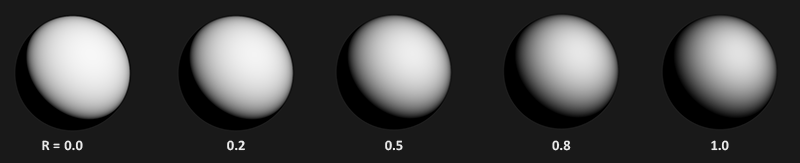The geometry function is a multiplier between [0.0, 1.0] with 1.0 (or white) measuring no microfacet shadowing, and 0.0 (or black) complete microfacet shadowing.

In GLSL the geometry function translates to the following code:


float GeometrySchlickGGX(float NdotV, float k)
{
float nom   = NdotV;
float denom = NdotV * (1.0 - k) + k;

return nom / denom;
}

float GeometrySmith(vec3 N, vec3 V, vec3 L, float k)
{
float NdotV = max(dot(N, V), 0.0);
float NdotL = max(dot(N, L), 0.0);
float ggx1 = GeometrySchlickGGX(NdotV, k);
float ggx2 = GeometrySchlickGGX(NdotL, k);

return ggx1 * ggx2;
}


### Fresnel equation

The Fresnel equation (pronounced as Freh-nel) describes the ratio of light that gets reflected over the light that gets refracted, which varies over the angle we're looking at a surface. The moment light hits a surface, based on the surface-to-view angle, the Fresnel equation tells us the percentage of light that gets reflected. From this ratio of reflection and the energy conservation principle we can directly obtain the refracted portion of light.

Every surface or material has a level of base reflectivity when looking straight at its surface, but when looking at the surface from an angle all reflections become more apparent compared to the surface's base reflectivity. You can check this for yourself by looking at your (presumably) wooden/metallic desk which has a certain level of base reflectivity from a perpendicular view angle, but by looking at your desk from an almost 90 degree angle you'll see the reflections become much more apparent. All surfaces theoretically fully reflect light if seen from perfect 90-degree angles. This phenomenon is known as Fresnel and is described by the Fresnel equation.

The Fresnel equation is a rather complex equation, but luckily the Fresnel equation can be approximated using the Fresnel-Schlick approximation:

$F_{Schlick}(h, v, F_0) = F_0 + (1 - F_0) ( 1 - (h \cdot v))^5$

$$F_0$$ represents the base reflectivity of the surface, which we calculate using something called the indices of refraction or IOR. As you can see on a sphere surface, the more we look towards the surface's grazing angles (with the halfway-view angle reaching 90 degrees), the stronger the Fresnel and thus the reflections: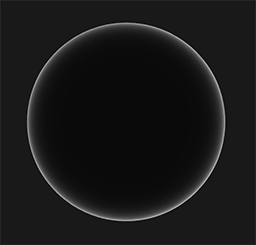There are a few subtleties involved with the Fresnel equation. One is that the Fresnel-Schlick approximation is only really defined for dielectric or non-metal surfaces. For conductor surfaces (metals), calculating the base reflectivity with indices of refraction doesn't properly hold and we need to use a different Fresnel equation for conductors altogether. As this is inconvenient, we further approximate by pre-computing the surface's response at normal incidence ($$F_0$$) at a 0 degree angle as if looking directly onto a surface. We interpolate this value based on the view angle, as per the Fresnel-Schlick approximation, such that we can use the same equation for both metals and non-metals.

The surface's response at normal incidence, or the base reflectivity, can be found in large databases like these with some of the more common values listed below as taken from Naty Hoffman's course notes:

Material $$F_0$$ (Linear) $$F_0$$ (sRGB) Color
Water (0.02, 0.02, 0.02)  (0.15, 0.15, 0.15)
Plastic / Glass (Low) (0.03, 0.03, 0.03) (0.21, 0.21, 0.21)
Plastic High (0.05, 0.05, 0.05) (0.24, 0.24, 0.24)
Glass (high) / Ruby (0.08, 0.08, 0.08) (0.31, 0.31, 0.31)
Diamond (0.17, 0.17, 0.17) (0.45, 0.45, 0.45)
Iron (0.56, 0.57, 0.58) (0.77, 0.78, 0.78)
Copper (0.95, 0.64, 0.54) (0.98, 0.82, 0.76)
Gold (1.00, 0.71, 0.29) (1.00, 0.86, 0.57)
Aluminium (0.91, 0.92, 0.92) (0.96, 0.96, 0.97)
Silver (0.95, 0.93, 0.88) (0.98, 0.97, 0.95)

What is interesting to observe here is that for all dielectric surfaces the base reflectivity never gets above 0.17 which is the exception rather than the rule, while for conductors the base reflectivity starts much higher and (mostly) varies between 0.5 and 1.0. Furthermore, for conductors (or metallic surfaces) the base reflectivity is tinted. This is why $$F_0$$ is presented as an RGB triplet (reflectivity at normal incidence can vary per wavelength); this is something we only see at metallic surfaces.

These specific attributes of metallic surfaces compared to dielectric surfaces gave rise to something called the metallic workflow. In the metallic workflow we author surface materials with an extra parameter known as metalness that describes whether a surface is either a metallic or a non-metallic surface.

Theoretically, the metalness of a material is binary: it's either a metal or it isn't; it can't be both. However, most render pipelines allow configuring the metalness of a surface linearly between 0.0 and 1.0. This is mostly because of the lack of material texture precision. For instance, a surface having small (non-metal) dust/sand-like particles/scratches over a metallic surface is difficult to render with binary metalness values.

By pre-computing $$F_0$$ for both dielectrics and conductors we can use the same Fresnel-Schlick approximation for both types of surfaces, but we do have to tint the base reflectivity if we have a metallic surface. We generally accomplish this as follows:


vec3 F0 = vec3(0.04);
F0      = mix(F0, surfaceColor.rgb, metalness);


We define a base reflectivity that is approximated for most dielectric surfaces. This is yet another approximation as $$F_0$$ is averaged around most common dielectrics. A base reflectivity of 0.04 holds for most dielectrics and produces physically plausible results without having to author an additional surface parameter. Then, based on how metallic a surface is, we either take the dielectric base reflectivity or take $$F_0$$ authored as the surface color. Because metallic surfaces absorb all refracted light they have no diffuse reflections and we can directly use the surface color texture as their base reflectivity.

In code, the Fresnel Schlick approximation translates to:


vec3 fresnelSchlick(float cosTheta, vec3 F0)
{
return F0 + (1.0 - F0) * pow(1.0 - cosTheta, 5.0);
}


With cosTheta being the dot product result between the surface's normal $$n$$ and the halfway $$h$$ (or view $$v$$) direction.

### Cook-Torrance reflectance equation

With every component of the Cook-Torrance BRDF described, we can include the physically based BRDF into the now final reflectance equation:

$L_o(p,\omega_o) = \int\limits_{\Omega} (k_d\frac{c}{\pi} + k_s\frac{DFG}{4(\omega_o \cdot n)(\omega_i \cdot n)}) L_i(p,\omega_i) n \cdot \omega_i d\omega_i$

This equation is not fully mathematically correct however. You may remember that the Fresnel term $$F$$ represents the ratio of light that gets reflected on a surface. This is effectively our ratio $$k_s$$, meaning the specular (BRDF) part of the reflectance equation implicitly contains the reflectance ratio $$k_s$$. Given this, our final final reflectance equation becomes:

$L_o(p,\omega_o) = \int\limits_{\Omega} (k_d\frac{c}{\pi} + \frac{DFG}{4(\omega_o \cdot n)(\omega_i \cdot n)}) L_i(p,\omega_i) n \cdot \omega_i d\omega_i$

This equation now completely describes a physically based render model that is generally recognized as what we commonly understand as physically based rendering, or PBR. Don't worry if you didn't yet completely understand how we'll need to fit all the discussed mathematics together in code. In the next chapters, we'll explore how to utilize the reflectance equation to get much more physically plausible results in our rendered lighting and all the bits and pieces should slowly start to fit together.

## Authoring PBR materials

With knowledge of the underlying mathematical model of PBR we'll finalize the discussion by describing how artists generally author the physical properties of a surface that we can directly feed into the PBR equations. Each of the surface parameters we need for a PBR pipeline can be defined or modeled by textures. Using textures gives us per-fragment control over how each specific surface point should react to light: whether that point is metallic, rough or smooth, or how the surface responds to different wavelengths of light.

Below you'll see a list of textures you'll frequently find in a PBR pipeline together with its visual output if supplied to a PBR renderer: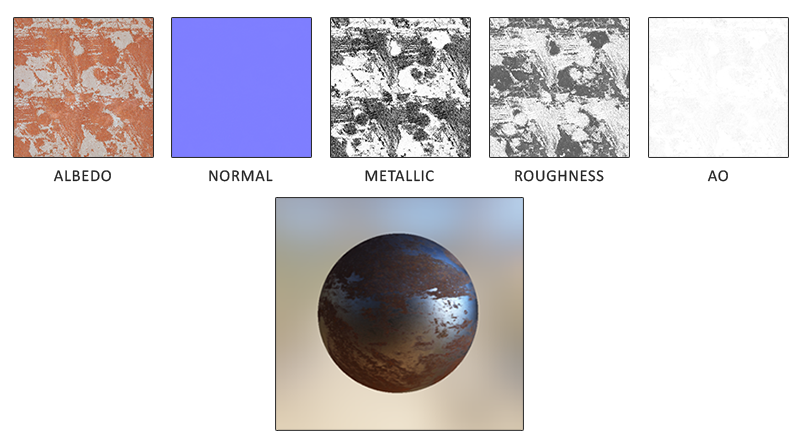Albedo: the albedo texture specifies for each texel the color of the surface, or the base reflectivity if that texel is metallic. This is largely similar to what we've been using before as a diffuse texture, but all lighting information is extracted from the texture. Diffuse textures often have slight shadows or darkened crevices inside the image which is something you don't want in an albedo texture; it should only contain the color (or refracted absorption coefficients) of the surface.

Normal: the normal map texture is exactly as we've been using before in the normal mapping chapter. The normal map allows us to specify, per fragment, a unique normal to give the illusion that a surface is bumpier than its flat counterpart.

Metallic: the metallic map specifies per texel whether a texel is either metallic or it isn't. Based on how the PBR engine is set up, artists can author metalness as either grayscale values or as binary black or white.

Roughness: the roughness map specifies how rough a surface is on a per texel basis. The sampled roughness value of the roughness influences the statistical microfacet orientations of the surface. A rougher surface gets wider and blurrier reflections, while a smooth surface gets focused and clear reflections. Some PBR engines expect a smoothness map instead of a roughness map which some artists find more intuitive. These values are then translated (1.0 - smoothness) to roughness the moment they're sampled.

AO: the ambient occlusion or AO map specifies an extra shadowing factor of the surface and potentially surrounding geometry. If we have a brick surface for instance, the albedo texture should have no shadowing information inside the brick's crevices. The AO map however does specify these darkened edges as it's more difficult for light to escape. Taking ambient occlusion in account at the end of the lighting stage can significantly boost the visual quality of your scene. The ambient occlusion map of a mesh/surface is either manually generated, or pre-calculated in 3D modeling programs.

Artists set and tweak these physically based input values on a per-texel basis and can base their texture values on the physical surface properties of real-world materials. This is one of the biggest advantages of a PBR render pipeline as these physical properties of a surface remain the same, regardless of environment or lighting setup, making life easier for artists to get physically plausible results. Surfaces authored in a PBR pipeline can easily be shared among different PBR render engines, will look correct regardless of the environment they're in, and as a result look much more natural.

HI